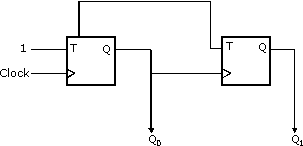Related Articles

# GATE | GATE CS 2010 | Question 65

• Difficulty Level : Medium
• Last Updated : 19 Nov, 2018

In the sequential circuit shown below,if the initial value of the output Q1Q0 is 00,what are the next four values of Q1Q0?(A) 11, 10, 01, 00
(B) 10, 11, 01, 00
(C) 10, 00, 01, 11
(D) 11, 10, 00, 01

Explanation: We have t flip flop

Truth table of t flip flop

 t q 0 q 1 q’

So q0 always inverted as t=1 always

So 1)q10=1

2)q0 =0

3)q0 =1

4)q0 =1

For q1 also t=1 always but clock is so we have to observe positive edge of clock I.e. when is q0 going from 0 -> 1

1)q1 =1

2)q1 =1

3)q1 =0

4)q1 =0

So final combination q0q1->(11,10,01,00) Ans (A)

Quiz of this Question

Attention reader! Don’t stop learning now.  Practice GATE exam well before the actual exam with the subject-wise and overall quizzes available in GATE Test Series Course.

Learn all GATE CS concepts with Free Live Classes on our youtube channel.

My Personal Notes arrow_drop_up